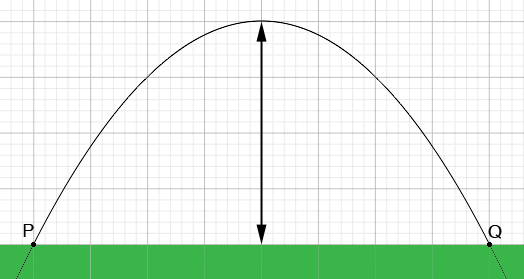SEARCH HOMEMath Central Quandaries & QueriesQuestion from Angela, a student: A parabolic arch has an equation of x^2 + 20y - 400 = 0 where x is measured in feet. How do I find the maximum height of the arch?Hi Angela,

A parabolic arch is symmetric around the line through its vertex.P and Q are the points where the graph crosses the x-axis. Find the x-coordinates of P and Q and then find the point on the x-axis half way between them. The maximum height of the arch occurs at this point.

PennyMath Central is supported by the University of Regina and The Pacific Institute for the Mathematical Sciences.